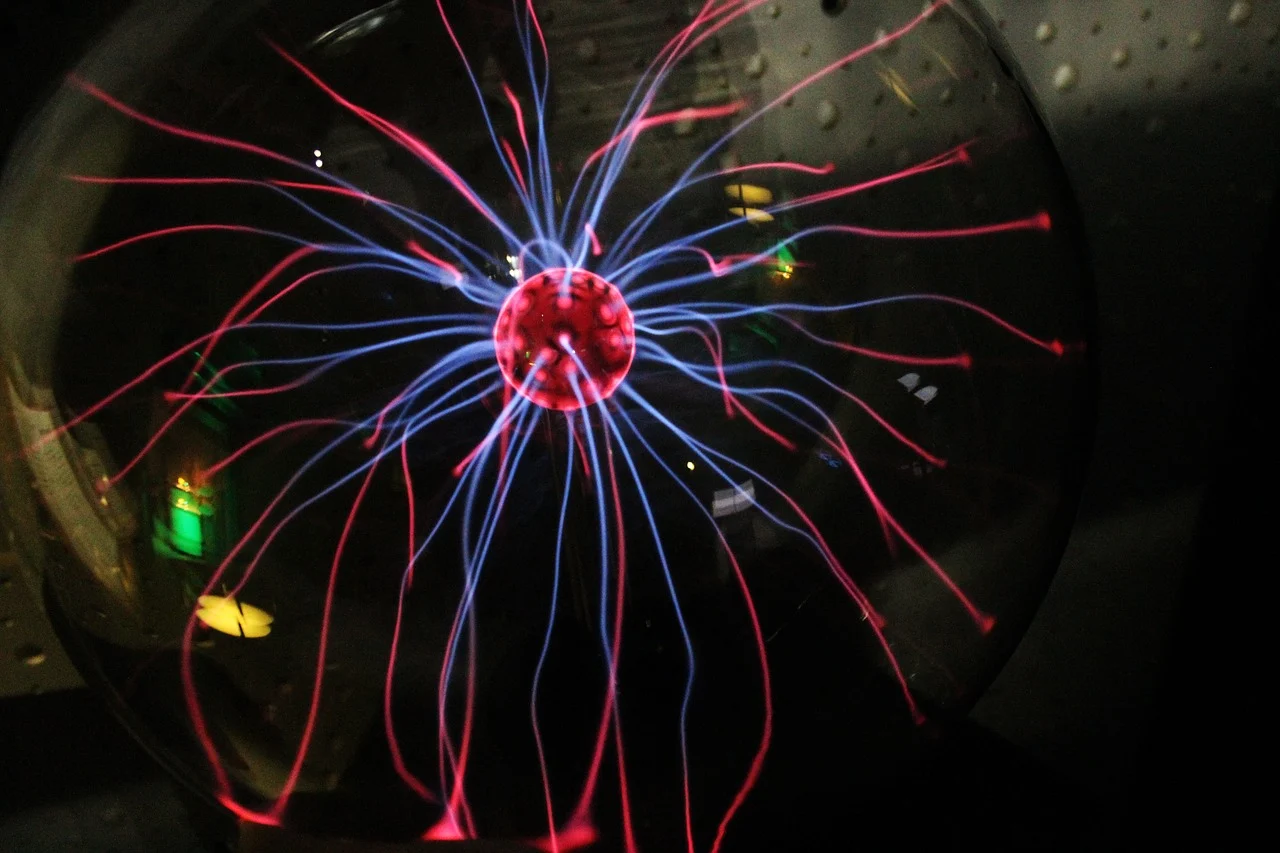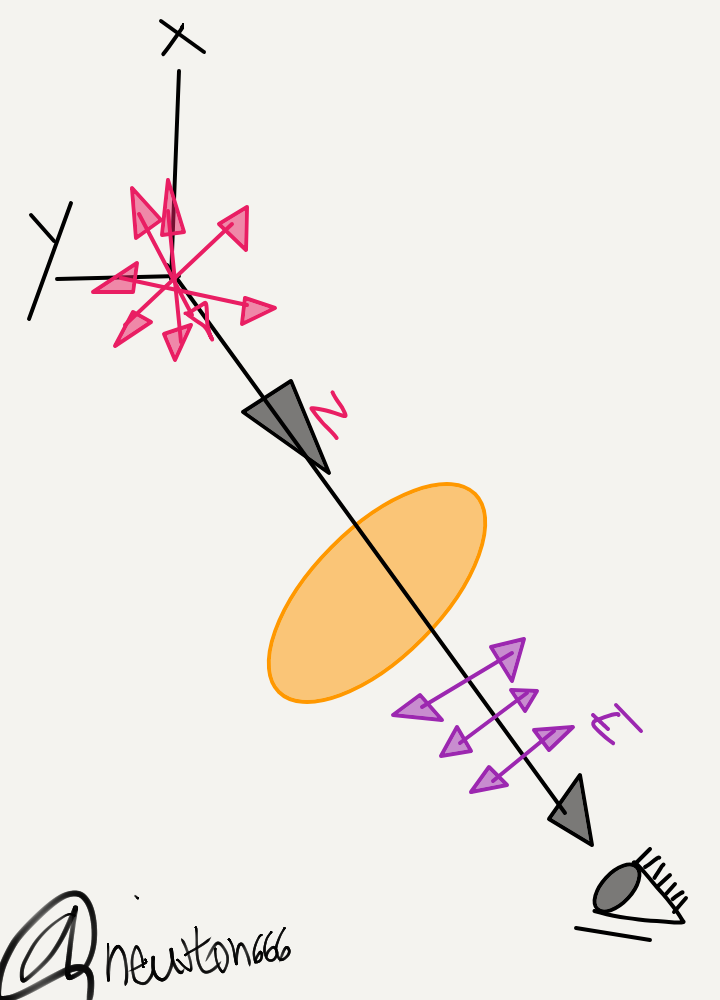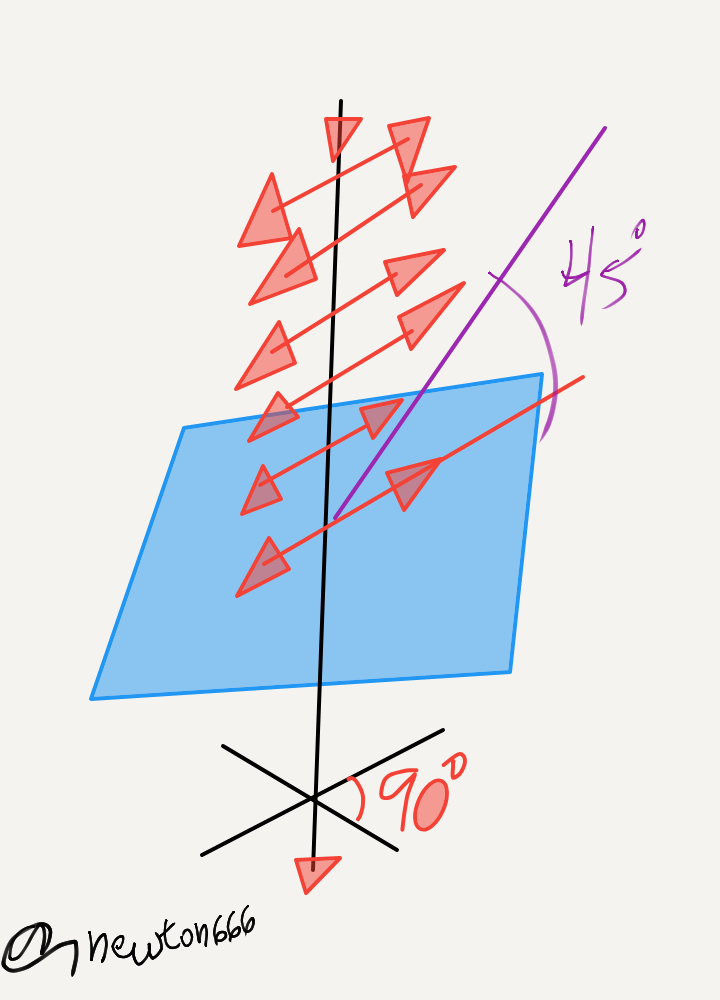# Electromagnetic wavesImage source pixabay

Greetings colleagues from the steemit community, welcome back to my blog, where you can find content from the wide universe of science, mathematics, biology, physics, chemistry, philosophy and general knowledge accompanied by scientific dissemination, a special salute to the communities that stimulates the content of science.

Continuing with the wave cycle in the field of physics, this time I bring you a very important type of waves which is characterized, dear reader, it is because of how it propagates without having a support or some axis of material support we are talking about electromagnetic waves, a very curious fact in the field of science such as the art of the history of mankind in 1865, James Maxwell in his studies and essays predicted the existence of this phenomenon, then Heinrich Hertz, affirmed this phenomenon based on the equation established by Maxwell, where it was shown that it could propagate at a speed of 300,000 km / s and that it is accompanied by vibration as part of an electric field.

E = E0. cos (ᦍ. t-k.z)

B = B0. cos (ᦍ. t-k.z)

According to these equations they are together and you will not be, since both have vibrations in plane perpendicular to the direction of their propagation. That is why light is considered a type of electromagnetic radiation visible to the human eye, thanks also to the fact that it is a type of variable electromagnetic field, where it exudes a combination of oscillating electric and magnetic fields, which are propagated through the space transporting energy from one place to another.

For the case of polarization in this part we find that the electromagnetic waves in the light characteristic, this are transverse waves where the direction of the disturbance is always perpendicular to the direction of propagation which in turn can be polarized, according to the axis In Z, there are infinite directions for the disturbance, although it should always be contained in a good plane, be it X, Y. An electric field in E with a constant direction is also evidenced, to verify this wave phenomenon by placing a sheet of polarized material, which has the very unique properties of eliminating all vibrations, only those that have a place in the plane that you want to study.Dear reader after knowing this effect in the experiment of the sheet, the circular and elliptical polarization is also found, it is attributed properties that some substance contains as part of its phenomena, such as quartz, calcite, cider, Because it is anisotropic optical characteristics, which the speed of light varies according to the direction of propagation of the wave. There is the case whereby using a polarized sheet two rays with emergent characteristics are studied, where it is found in a polarized point under a plane scheme of vibrations perpendicular to each other, to obtain the circular polarized light a calcite plate with 45º was used , with the direction of said plate joining waves and forming an angle of 90º, to obtain a circular polarization in the diagram of the Z axis, we will have a resulting vector with a clockwise direction.In this type of wave, frequency changes are found with relative movements depending on the source that produces the band and with respect to an observer, this phenomenon is Doppler from an emitter focus at rest and the observer, it should be considered that Electromagnetic radiation can manifest itself in various ways such as radio waves, microwaves, infrared radiation, visible light, ultraviolet radiation, X-rays, and gamma rays. A very unique piece of information is that the full range of wavelengths is what is called the electromagnetic spectrum.

What fascinates most about this topic, dear reader, is that electromagnetic waves start with a charged particle, where this particle creates an electric field that exerts a force on other particles, that is why they propagate in material media and in the vacuum, which at the same time has the characteristic of the direction of propagation is perpendicular to the direction of oscillation. For the case of an observer of fixed form, where the frequency would be ѵ = v / λ, where v is the propagation speed of the wave, but if the observer is evidence of a distance from the speed v0, this allows the wave to exert a velocity v + v0:

ѵ '= v + v0 / λ

ѵ '= ѵ (1 + v0 / v), for the case if it approaches it would be = ѵ (1 - v0 / v).